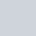in

# Oracle Apex: Inserting Records into Interactive Grid Using JavaScript

Below is an example to insert records into the interactive grid using the JavaScript in Oracle Apex.

To demonstrate the following example, I have created an interactive grid based on the following table:

```Create Table demo_js_insert (
code_seq    Number,
code_desc   Varchar2(20)
)
/```

Also, I have these non-database items on my page:

• P2_START_SEQ
• P2_END_SEQ
• P2_SEQ_DESC

### JavaScript Code Example

Created a button named "InsRecords", on which I have written the following JavaScript code to insert records into the interactive grid having the static id "ig_js_insert". I will set the field `code_seq` value to `P2_START_SEQ` value and will insert the records until the `P2_END_SEQ` value. Also, will set the value of a field `code_desc` to the `P2_SEQ_DESC` value.

```//change the ig_js_insert with the static id of your interactive grid
var widget      = apex.region('ig_js_insert').widget();
var grid        = widget.interactiveGrid('getViews','grid');
var model       = grid.model;
var n_code_start, n_code_end, v_desc;

n_code_start = parseInt(\$v("P2_START_SEQ"), 10);
n_code_end = parseInt(\$v("P2_END_SEQ"), 10);
v_desc = \$v("P2_SEQ_DESC");

for (let i = n_code_start; i <= n_code_end; i++) {

//insert new record on a model
var myNewRecordId = model.insertNewRecord();

//get the new record
var myNewRecord = model.getRecord(myNewRecordId);

//update record values
model.setValue(myNewRecord, 'CODE_SEQ', i.toString());
model.setValue(myNewRecord, 'CODE_DESC', v_desc);

}```

#### OutputAfter inserting the records, when the user will click on the Save button, then it will save it to the database table.

Reference:

Related tutorials: